# The rolling disk

Here, we explore the dynamics of a thin disk rolling without slipping over a rough horizontal surface. Analysis of the thin disk’s motion has a long history, dating back to the works of Appell , Chaplygin , and Korteweg  in the late 19th century.

Contents

## Equations of motion

Consider a thin axisymmetric disk with massand radiusthat rolls without slipping over a stationary and rough horizontal plane, as illustrated in Figure 1. We locate the disk’s mass center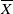using a set of Cartesian coordinates,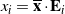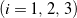, where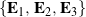is the space-fixed basis, and thus the disk’s linear momentum.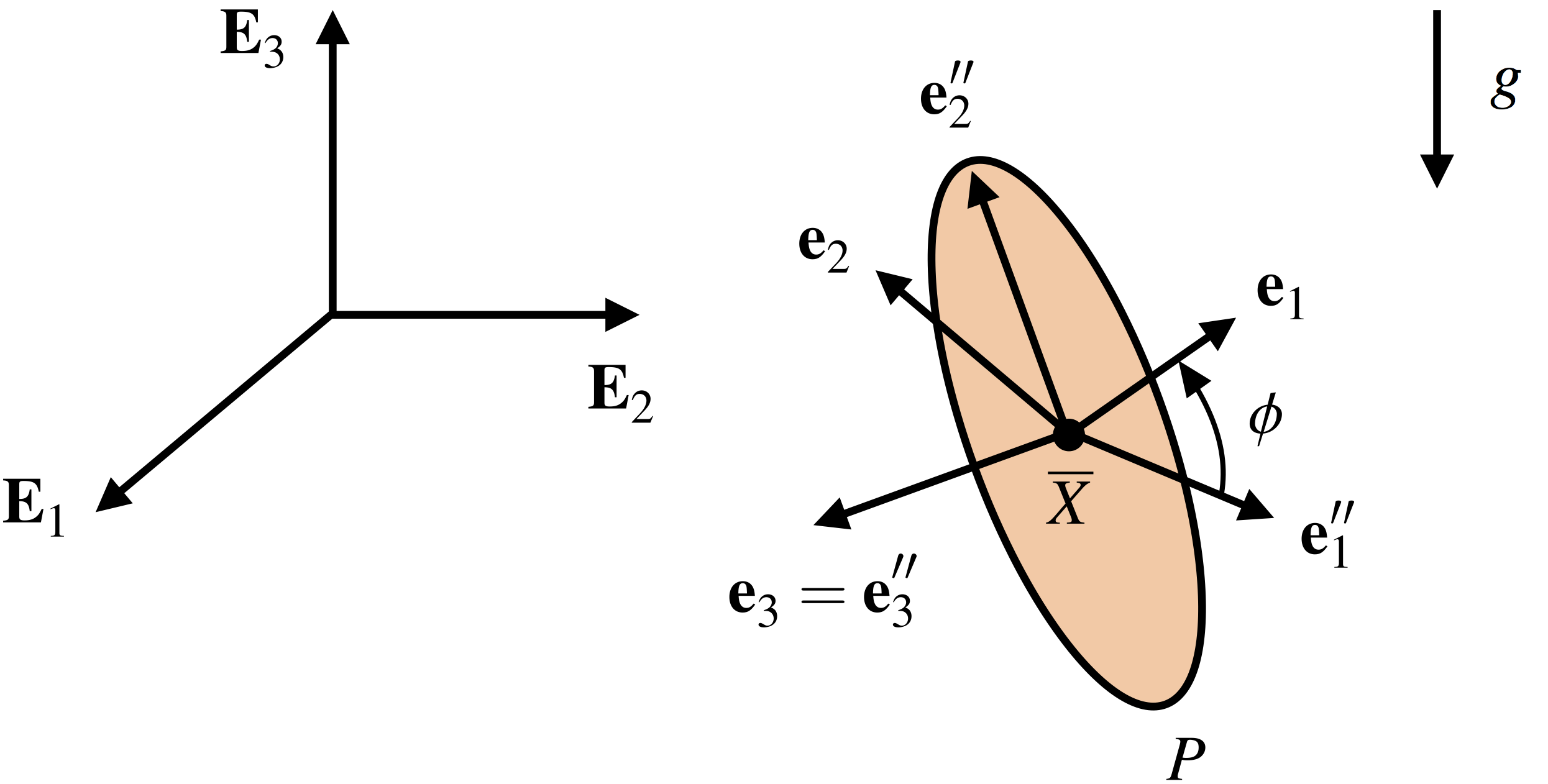Figure 1. Schematic of a thin rolling disk illustrating the alignment of the corotational basis . The basis , which is attached to the disk but not spinning with it, more conveniently describes the location of the disk’s instantaneous point of contact with the horizontal surface. The bases and are related by a spin about the direction.

The orientation of the disk is parameterized by set of Euler angles: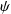,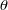, and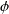. We assume the disk’s reference configuration is such that the disk lays flat in the horizontal plane, and therefore the disk’s spin axis corresponds to the corotational basis vector. The disk’s instantaneous point of contact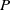with the surface is located relative to the center of mass according to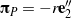, where the basis vector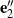is attached to the disk but not spinning with it, i.e., the basisand the corotational basis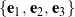are separated by a spinabout the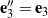direction.

Because the disk is axisymmetric, its principal moments of inertiaand. The angular momentum of the disk about its mass center is then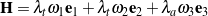, where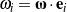denote the corotational components of the disk’s angular velocity. Using a 3-1-3 set of Euler angles,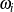are related to,, andand their rates of change as follows:

(1)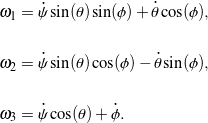The disk is subject to three constraints arising from the fact that the instantaneous point of contactwith the fixed horizontal surface must have zero velocity for the disk to roll without slipping:. One of these constraints,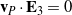(that is, the disk maintains contact with the surface), is integrable (i.e., holonomic), while the remaining two constraints,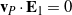and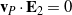, are non-integrable (or non-holonomic).

We may obtain the disk’s equations of motion by applying a balance of linear momentum and a balance of angular momentum with respect to the disk’s mass center: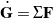and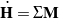, respectively. The disk’s weight, the vertical reaction force exerted by the surface on the bottom of the disk, and the static friction force acting at the instantaneous contact pointcomprise the net force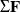and contribute to the net moment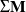about the disk’s center of mass. The resulting equations of motion from applying the balance laws coupled with the non-integrable constraints form a set of differential equations that describe the disk’s orientation and the lateral translation of its mass center over time. We may conveniently express this system of equations in the first-order formsuitable for numerical integration in MATLAB. Taking the state vector, we have

(2)## Simulation and animation

Assuming the horizontal surface is sufficiently rough to prevent slipping, a typical simulated rolling motion of the disk is animated in Figure 2, where we trace out the path of the instantaneous point of contactwith the surface to further highlight the disk’s behavior.

The disk is able to execute a steady motion in which it rolls at a constant rate in a circular path. This motion is possible if the disk’s precession rate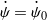, nutation angle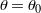, and spin rate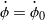are constant and satisfy the condition

(3)A sample steady motion of the disk is shown in Figure 3.

Because the instantaneous point of contactwith the horizontal surface has zero velocity, the static friction force acting on the disk does no work, and thus the disk’s total mechanical energy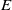is conserved:

(4)Surprisingly, the rolling disk has associated with it two other conserved quantities that were discovered in the late 19th century by Appell , Chaplygin , and Korteweg  (see [4, 5, 6, 7]). However, their physical interpretation is still an open question.

Finally, we may extend our analysis of the rolling disk to very simply demonstrate the counterintuitive nature of making a turn on a bicycle or motorcycle: to turn right, for example, one must push forward on the right handlebar. Suppose the disk now includes a very light axle that passes through the disk’s center along its spin axis. We can cause the disk to initiate a right turn by exerting a forward forceon the right side of the axle at a distance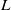from the disk’s center. An animation of this simulated behavior is provided in Figure 4. It is important to note that the forceis applied throughout the duration of the simulation, causing the disk to accelerate and travel in an outward spiraling path.